# Math for Data Science

Learning PathSkills: Statistics, Correlation, Linear Regression, Logistic Regression

In this learning path, you’ll gain the mathematical foundations you’ll need to get ahead with data science.

## Math for Data Science

Learning Path ⋅ 5 Resources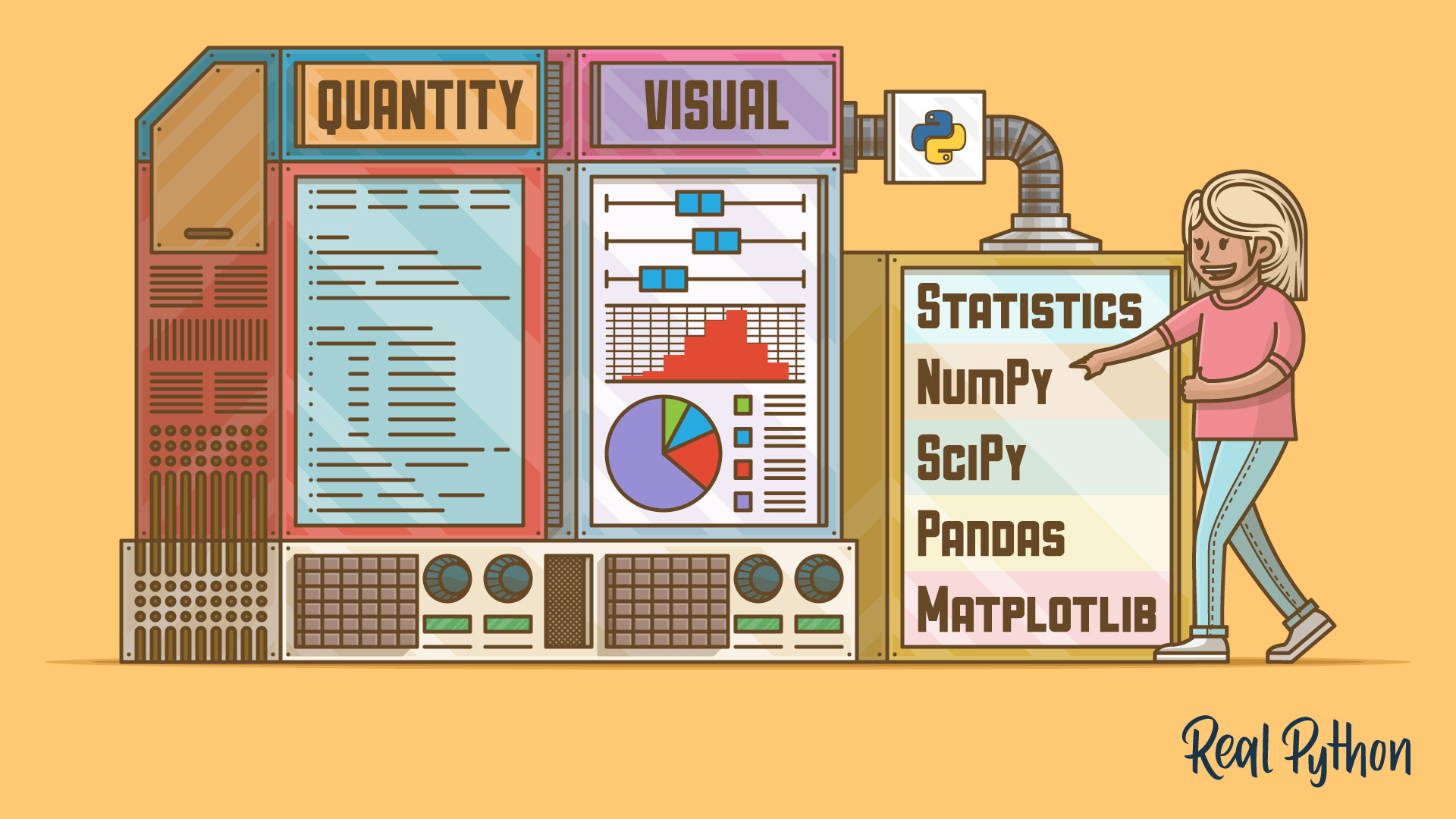Tutorial

### Python Statistics Fundamentals: How to Describe Your Data

Learn the fundamentals of descriptive statistics and how to calculate them in Python. You'll find out how to describe, summarize, and represent your data visually using NumPy, SciPy, pandas, Matplotlib, and the built-in Python statistics library.

#1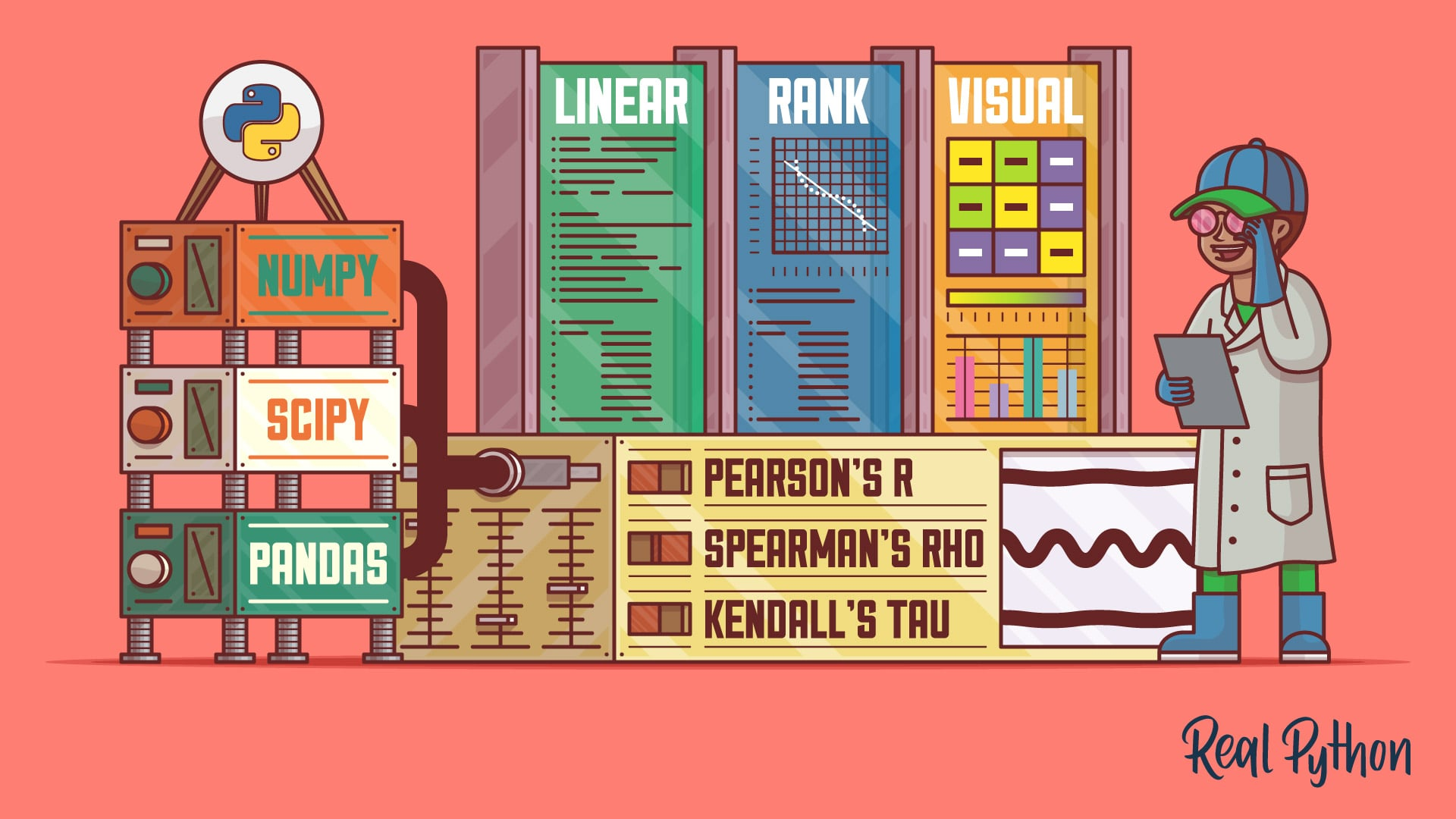Tutorial

### NumPy, SciPy, and pandas: Correlation With Python

Learn what correlation is and how you can calculate it with Python. You'll use SciPy, NumPy, and pandas correlation methods to calculate three different correlation coefficients. You'll also see how to visualize data, regression lines, and correlation matrices with Matplotlib.

#2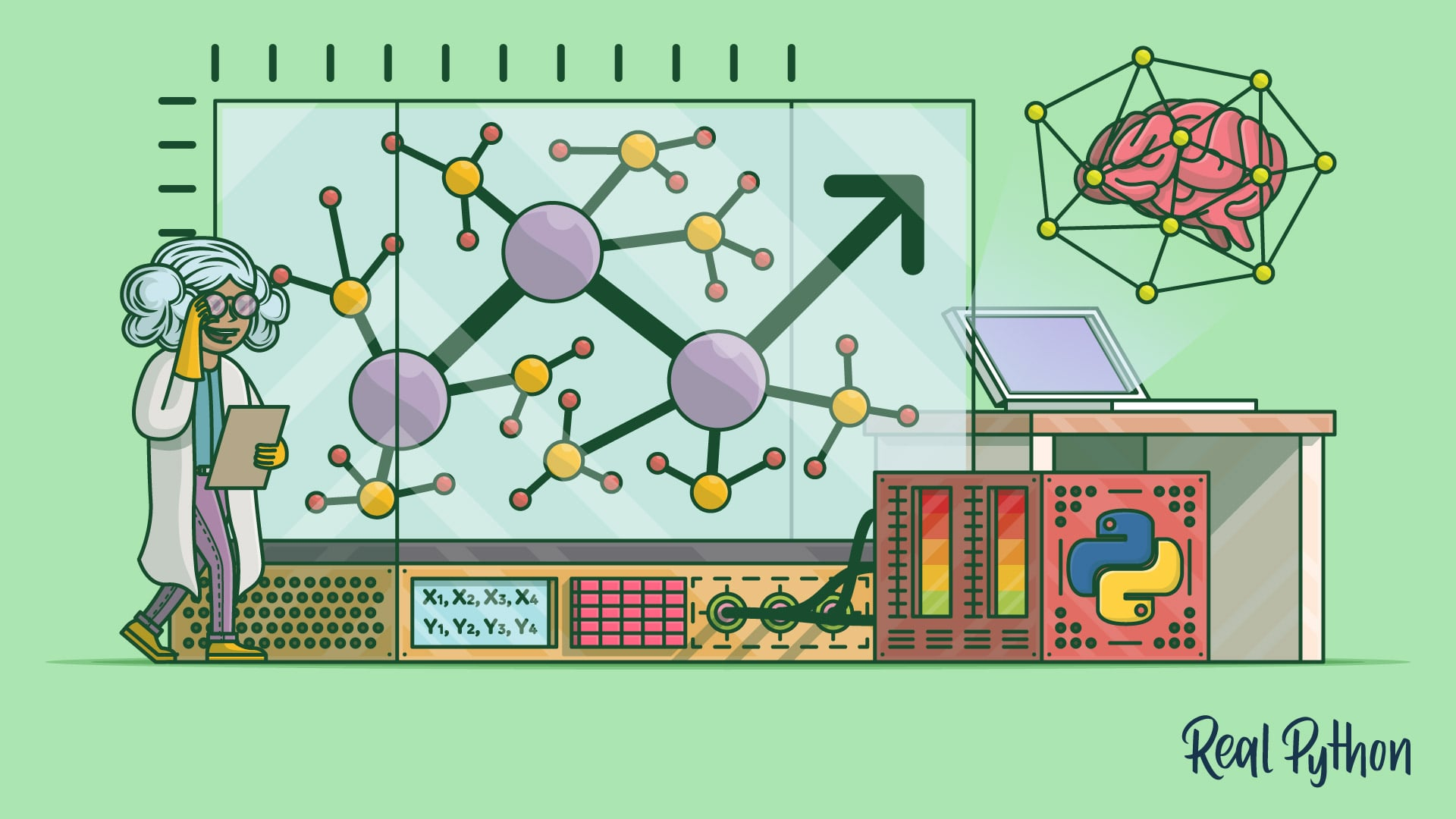Course

### Starting With Linear Regression in Python

In this video course, you'll get started with linear regression in Python. Linear regression is one of the fundamental statistical and machine learning techniques, and Python is a popular choice for machine learning.

#3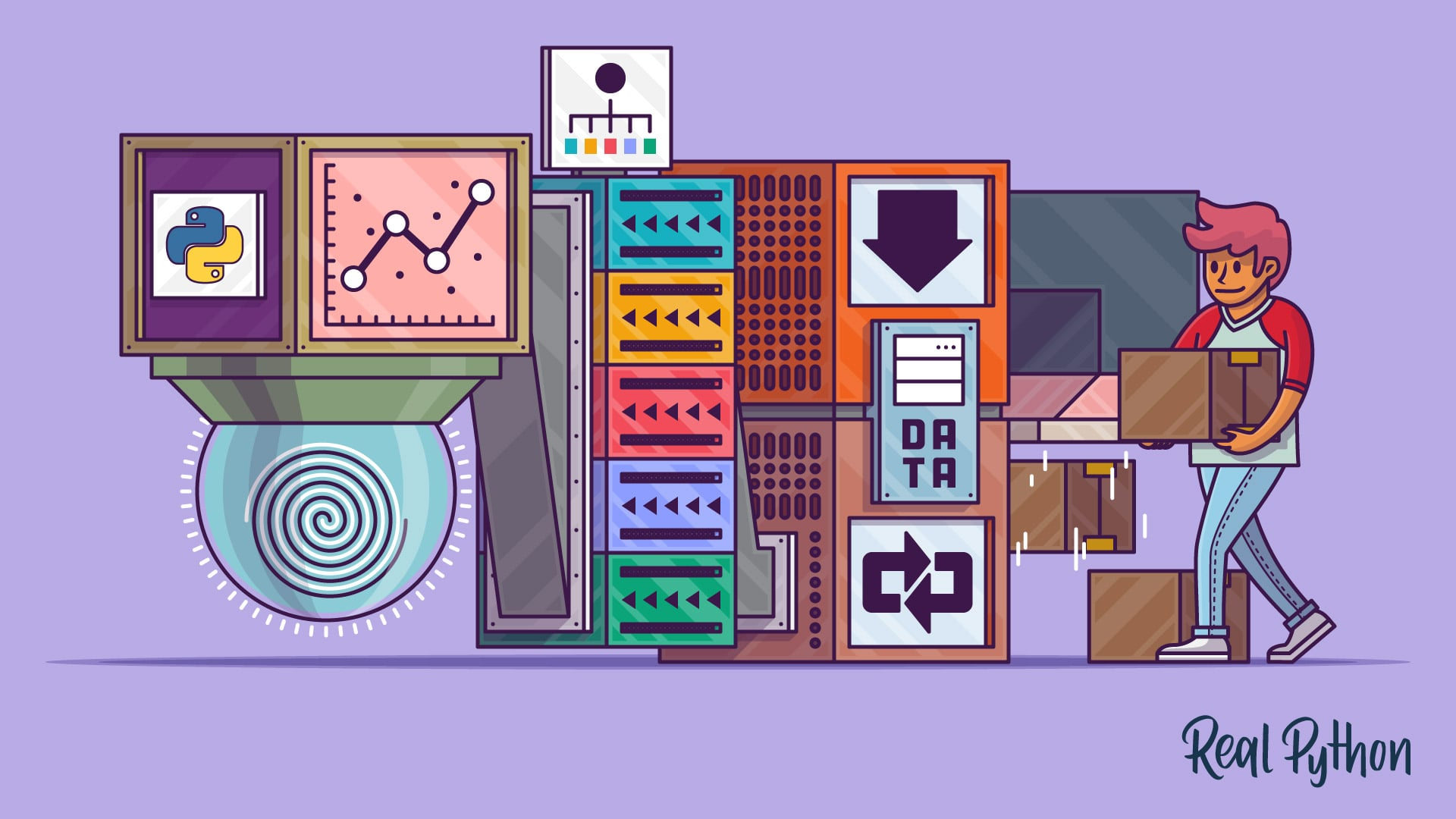Tutorial

### Logistic Regression in Python

Get started with logistic regression in Python. Classification is one of the most important areas of machine learning, and logistic regression is one of its basic methods. You'll learn how to create, evaluate, and apply a model to make predictions.

#4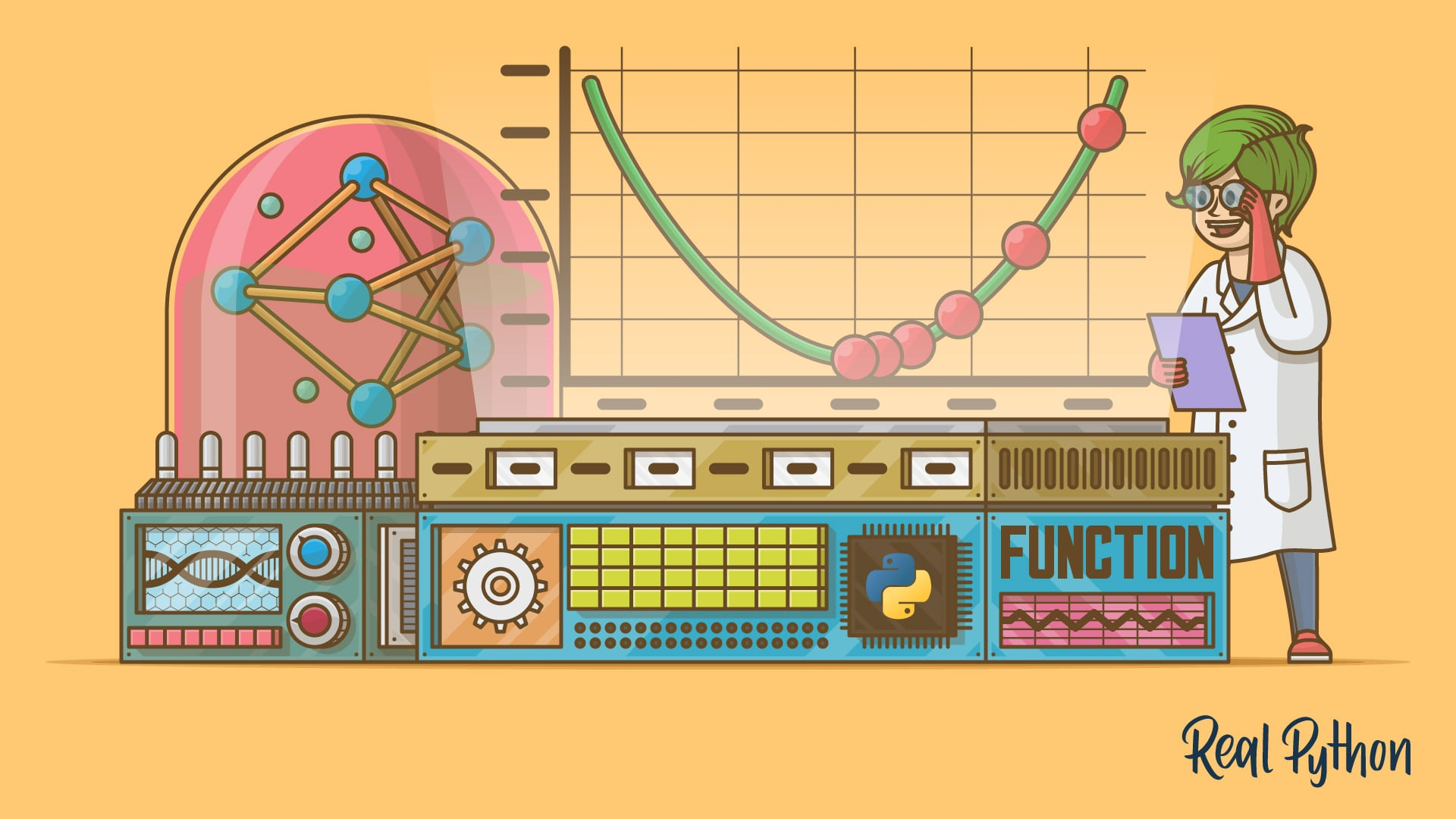Tutorial

### Stochastic Gradient Descent Algorithm With Python and NumPy

Learn what the stochastic gradient descent algorithm is, how it works, and how to implement it with Python and NumPy.

#5

Got feedback on this learning path?

Looking for real-time conversation? Visit the Real Python Community Chat or join the next “Office Hours” Live Q&A Session. Happy Pythoning!

« Browse All Learning Paths